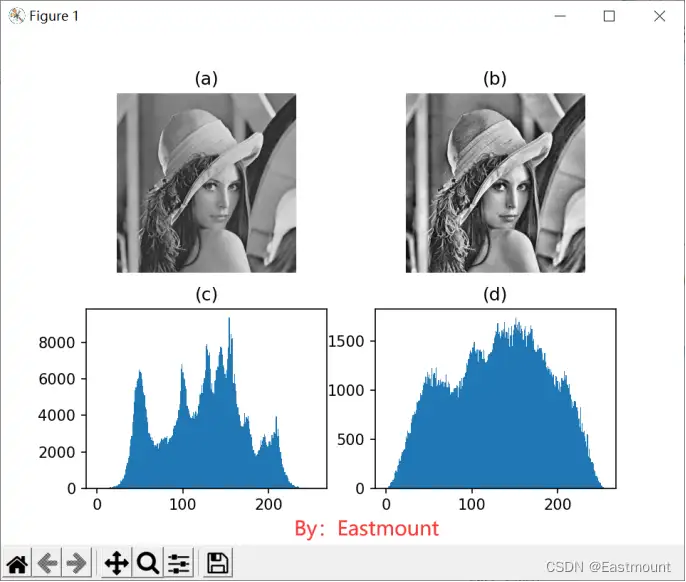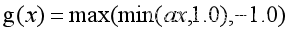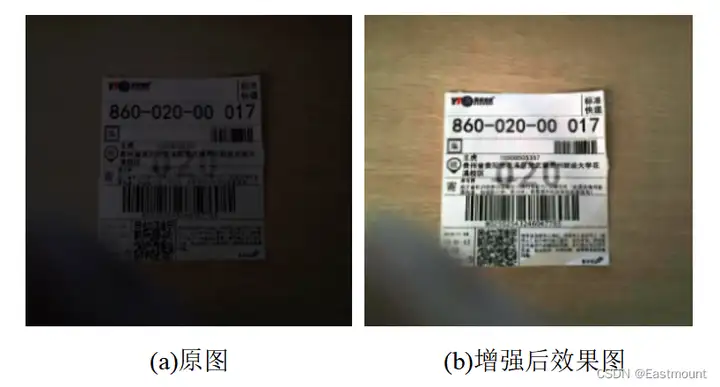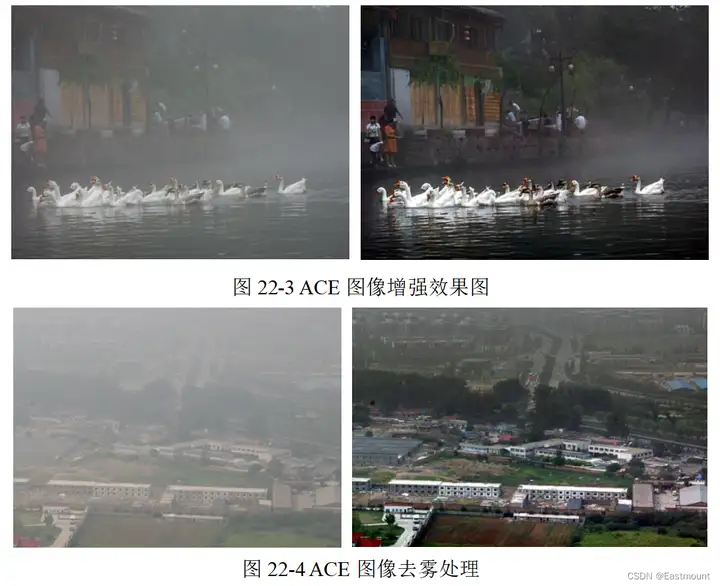﻿ 跟我學Python丨圖像增強及運算：局部直方圖均衡化和自動色彩均衡化處理_ZenDei技術網路在線

# 跟我學Python丨圖像增強及運算：局部直方圖均衡化和自動色彩均衡化處理## 一.局部直方圖均衡化

retval = createCLAHE([, clipLimit[, tileGridSize]])

• clipLimit參數表示對比度的大小
• tileGridSize參數表示每次處理塊的大小

```# -*- coding: utf-8 -*-
# By:Eastmount
import cv2
import numpy as np
import matplotlib.pyplot as plt
#讀取圖片
#灰度轉換
gray = cv2.cvtColor(img, cv2.COLOR_BGR2GRAY)
#局部直方圖均衡化處理
clahe = cv2.createCLAHE(clipLimit=2, tileGridSize=(10,10))
#將灰度圖像和局部直方圖相關聯, 把直方圖均衡化應用到灰度圖
result = clahe.apply(gray)
#顯示圖像
plt.subplot(221)
plt.imshow(gray, cmap=plt.cm.gray), plt.axis("off"), plt.title('(a)')
plt.subplot(222)
plt.imshow(result, cmap=plt.cm.gray), plt.axis("off"), plt.title('(b)')
plt.subplot(223)
plt.hist(img.ravel(), 256), plt.title('(c)')
plt.subplot(224)
plt.hist(result.ravel(), 256), plt.title('(d)')
plt.show()```## 二.自動色彩均衡化

Retinex演算法是代表性的圖像增強演算法，它根據人的視網膜和大腦皮層模擬對物體顏色的波長光線反射能力而形成，對複雜環境下的一維條碼具有一定範圍內的動態壓縮，對圖像邊緣有著一定自適應的增強。自動色彩均衡（Automatic Color Enhancement，ACE）演算法是在Retinex演算法的理論上提出的，它通過計算圖像目標像素點和周圍像素點的明暗程度及其關係來對最終的像素值進行校正，實現圖像的對比度調整，產生類似人體視網膜的色彩恆常性和亮度恆常性的均衡，具有很好的圖像增強效果[3-4]。

ACE演算法包括兩個步驟，一是對圖像進行色彩和空域調整，完成圖像的色差校正，得到空域重構圖像；二是對校正後的圖像進行動態擴展。ACE演算法計算公式如下：```# -*- coding: utf-8 -*-
# By:Eastmount
# 參考zmshy2128老師文章
import cv2
import numpy as np
import math
import matplotlib.pyplot as plt
#線性拉伸處理
#去掉最大最小0.5%的像素值 線性拉伸至[0,1]
def stretchImage(data, s=0.005, bins = 2000):
ht = np.histogram(data, bins);
d = np.cumsum(ht)/float(data.size)
lmin = 0; lmax=bins-1
while lmin<bins:
if d[lmin]>=s:
break
lmin+=1
while lmax>=0:
if d[lmax]<=1-s:
break
lmax-=1
return np.clip((data-ht[lmin])/(ht[lmax]-ht[lmin]), 0,1)
#根據半徑計算權重參數矩陣
g_para = {}
global g_para
if m is not None:
return m
m = np.zeros((size, size))
if h==0 and w==0:
continue
m /= m.sum()
return m
#常規的ACE實現
height,width = I.shape
#Python3報錯如下 使用列表append修改
zh = []
zw = []
n = 0
zh.append(0)
zw.append(0)
n += 1
for n in range(height):
zh.append(n)
for n in range(width):
zw.append(n)
n = 0
zh.append(height-1)
zw.append(width-1)
n += 1
#print(zh)
#print(zw)
Z = I[np.ix_(zh, zw)]
res = np.zeros(I.shape)
if para[h][w] == 0:
continue
res += (para[h][w] * np.clip((I-Z[h:h+height, w:w+width])*ratio, -1, 1))
return res
#單通道ACE快速增強實現
print(I)
height, width = I.shape[:2]
if min(height, width) <=2:
return np.zeros(I.shape)+0.5
Rs = cv2.resize(I, (int((width+1)/2), int((height+1)/2)))
Rf = zmIceFast(Rs, ratio, radius) #遞歸調用
Rf = cv2.resize(Rf, (width, height))
Rs = cv2.resize(Rs, (width, height))
res = np.zeros(I.shape)
for k in range(3):
return res
#主函數
if __name__ == '__main__':
res = zmIceColor(img/255.0)*255
cv2.imwrite('Ice.jpg', res)```## 三.總結• 下載jdk安裝包 進入Oracle官網進行下載：Java Downloads | Oracle 鏡像源也可以下載 為什麼配置環境變數 系統在運行命令和程式的時候先從當前目錄進行查找，找不到會去配置的環境變數裡面找 配置環境變數的目的是為了命令行在各個位置都能訪問 平時用IDEA集成開發工具不非得配置 ...
• 1 簡介 DB2是IBM的一款優秀的關係型資料庫，簡單學習一下。 2 Docker安裝DB2 為了快速啟動，直接使用Docker來安裝DB2。先下載鏡像如下： docker pull ibmcom/db2:11.5.0.0 啟動資料庫如下： docker run -itd \ --name mydb ...
• Spring管理Bean-IOC-02 2.基於XML配置bean 2.7通過util空間名稱創建list BookStore.java： package com.li.bean; import java.util.List; /** * @author 李 * @version 1.0 */ pu ...
• Spring6 Spring項目的創建 打開IDEA，新建一個maven項目 在maven項目中引入spring的倉庫和依賴 <repositories> <repository> <id>repository.spring.milestone</id> <name>Spring Milestone ...
• 2023-01-17 一、Spring中的註解 1、使用註解的原因 （1）使用註解將對象裝配到IOC容器中 （2）使用註解管理對象之間依賴關係（自動裝配） 2、Spring中裝配對象的註解 （1）@Component 標識一個受Spring IOC容器管理的普通組件 （2）@Repository 標 ...
• 好久沒有更新文章了，高齡開發沒什麼技術，去了外包公司後沒怎麼更新文章了。今天分享下統一處理starter，相信開發web系統的時候都是會涉及到前後端的交互，而後端返回數據的時候一般都會統一封裝一個返回對象和統一處理異常，一般情況下都是在controller的每個方法中調用封裝的對象，把相應的數據塞到 ...
• 2023-01-17 一、Spring管理druid步驟 （1）導入jar包 <!-- https://mvnrepository.com/artifact/com.alibaba/druid --> <dependency> <groupId>com.alibaba</groupId> <arti ...
• 抽獎程式 ''' 抽獎程式 使用時可以修改嘉賓名單，然後單機‘開始’和‘停止’按鈕 來控制界面上名單的滾動實現抽獎功能，涉及的模塊主要 有多線程 ''' import itertools import random import threading import time import tkinte ...